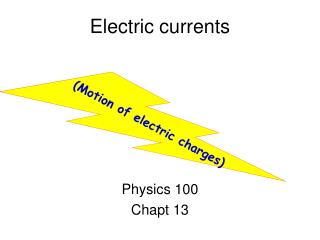# Electric currents - PowerPoint PPT PresentationDownload PresentationElectric currents

Electric currentsDownload Presentation## Electric currents

- - - - - - - - - - - - - - - - - - - - - - - - - - - E N D - - - - - - - - - - - - - - - - - - - - - - - - - - -
##### Presentation Transcript

1. Electric currents (Motion of electric charges) Physics 100 Chapt 13

2. Alessandro Volta

3. Positive Ions Atoms with one or more electrons removed _ _ + + + _ _ _ + + _ _ _ ”net” charge = +2qe

4. Battery C Zn Zn - - + + Zn Zn++ Zn++ Zn - - - - Zn Zn + + - - Zn++ - - Zn Zn Zn++ + + acid

5. “Voltage” Cathode Anode - + - - + + - - + + - E - + + - Zn++ - F + + W = Fd “Voltage” F = 2qeE d W0 2qe V W = 2qeEd  W0 = 2qeE0d  =E0d

6. Energy gained by the charge W = Fd =Q E0d = QV F=QE0 F=QE0 Q Q Anode - + Cathode - E0 - + + - - + + Zn++ - - + + - Zn++ - + + d

7. Units again! W = Q V joules joules coulomb W Q  V = = Volt coulombs joule coulomb 1 V = 1

8. Continuous charge flow = “electric current” Electrical “conductor” connected between anode & cathode Q Q Anode - + Cathode - - + + - - + + Zn++ - - + + - Zn++ - + +

9. electric current Coulombs second Units: Q t I = =Amperes Q Q Anode - + Cathode - - + + - - + + Zn++ - - + + - Zn++ - + +

10. The conductor can be a piece of wire Q t I = + + + Anode - + Cathode - - + + - - + + Zn++ - - + + - Zn++ - + +

11. The energy can be used to run a gadget Energy time QV t P= = = I V + I + + I I Anode - + Cathode - - + + - - + + Zn++ - - + + - Zn++ - + +

12. Electric light 60 Watts I=? T Power = P = I V P V 60 W 100V I = = J/s J/C 1/s 1/C = 0.6 = 0.6 C s V=100V = 0.6 = 0.6 A

13. General circuit I Appliance - + I + - 12V Energy source (device that separates + & - charge)

14. analogy Amt of water flow ~ current appliance Height ~ voltage Pump ~ battery pump pond